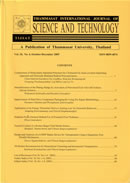THAMMASAT INTERNATIONAL JOURNAL OF SCIENCE & TECHNOLOGY

Volume 25, No. 01, Month JANUARY, Year 2020, Pages 128 - 141

Embedded pseudo-runge-kutta methods for first and second order initial value problems

Shruti Tiwari, Ram Kishor Pandey, Harendra Singh, Jagdev Singh

Abstract

In this paper, we have proposed an embedded pseudo-Runge-Kutta method (EPRKM) of order three. We have developed two implicit embedded pseudo Runge-Kutta methods (EPRKM) for the solution of first order initial value problems of which the first one is fully implicit and the other is diagonally implicit. Also, a two-step explicit embedded pseudo- Runge-Kutta method for solving second order initial value problems is also derived. This development is an effort to minimize the computational cost of classical Runge-Kutta (RK) method. A classical s-stage RK method requires s function evaluations (slopes) per step. The present methods require four evaluations in the first step and from the second step onwards it requires only two evaluations per step. The cost of computation in the form of number of function evaluations (slopes) are compared via Table 1, from which it is evident that our proposed developments are cost-efficient. The proposed methods are tested on two initial value problems (IVPs) and the errors obtained by these methods are compared with the errors by RK third order (RK3), third order implicit Runge-Kutta (IRK3) method and Runge-Kutta- Nyström 3-stage method (RKN3) which are shown in Tables 2 and 3. The convergence and stability properties are established and the governing theorems are also derived. Intervals and regions of absolute stability of the proposed methods are also derived, and regions are depicted in Fig. 1. The proposed methods have wide scope of applicability for the numerical solution of IVPs which are frequently occurred in physics, chemistry, molecular dynamics, fluid dynamics and several branches of engineering.

Keywords

Initial value problem, Interval of absolute stability, Pseudo Runge-Kutta method, Runge-Kutta method, Stability properties

THAMMASAT INTERNATIONAL JOURNAL OF SCIENCE & TECHNOLOGY